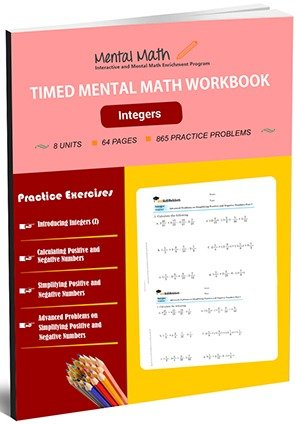13,953## Timed Integers Worksheets

Integers (…-3, -2, -1, 0, 1, 2, 3…) is the extended form of positive numbers (1, 2, 3,…) which are the image of positive numbers if a mirror is at zero. In the system of integers, subtraction operation can be performed. Students start integers section with adding and subtraction positive and negative numbers. Similarly, they multiply and divide positive and negative numbers. At the last, they simplify positive and negative numbers with combined four mathematical operations addition, subtraction, multiplication, and division.

Leave a Review

Worksheet # Topics/Curriculum # of Pages Sample
Unit-1 Introducing Integers (Z) 8 pagesUnit-2 Adding and Subtracting Positive and Negative Numbers 8 pagesUnit-3 Calculating Positive and Negative Numbers 8 pagesUnit-4 Multiplying Positive and Negative Numbers 8 pagesUnit-5 Dividing Positive and Negative Numbers 8 pagesUnit-6 Simplifying Positive and Negative Numbers 8 pagesUnit-7 More Problems on Simplifying Positive and Negative Numbers 8 pagesUnit-8 Advanced Problems on Simplifying Positive and Negative Numbers 8 pages